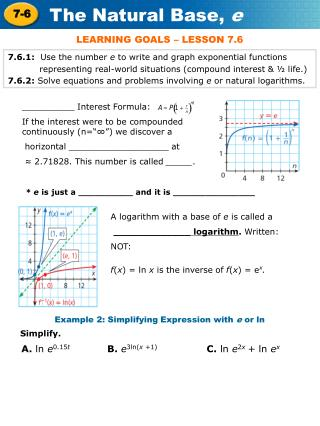DownloadDownload Presentation7.6.1: Use the number e to write and graph exponential functions

# 7.6.1: Use the number e to write and graph exponential functions

Download Presentation## 7.6.1: Use the number e to write and graph exponential functions

- - - - - - - - - - - - - - - - - - - - - - - - - - - E N D - - - - - - - - - - - - - - - - - - - - - - - - - - -
##### Presentation Transcript

1. LEARNING GOALS – LESSON 7.6 7.6.1: Use the number e to write and graph exponential functions representing real-world situations (compound interest & ½ life.) 7.6.2: Solve equations and problems involving e or natural logarithms. __________ Interest Formula: If the interest were to be compounded continuously (n=“∞”) we discover a horizontal ___________________ at ≈ 2.71828. This number is called _____. * e is just a __________ and it is _______________ A logarithm with a base of e is called a _____________ logarithm. Written: NOT: f(x) = ln x is the inverse of f(x) = ex. Example 2: Simplifying Expression with e or ln Simplify. A. ln e0.15t B. e3ln(x +1) C. ln e2x + ln ex

2. Check It Out! Example 2 Simplify. D. ln e3.2 E. e2lnx F.ln ex +4y Continuously Compounded Interest: A = Pert A – P – r – t – Example 3: Economics Application • What is the total amount for an investment of \$500 invested at 5.25% for 40 years and compounded continuously? • If the total amount for an investment of \$500 invested for 50 years and compounded continuously was \$10,000 what was the interest rate? A = Pert A = Pert

3. Half-Life of a Substance: N(t) = N0e-kt N(t) – N0 – k – t – Example 4: Science Application A. Pluonium-239 (Pu-239) has a half-life of 24,110 years. How long does it take for a 1 g sample of Pu-239 to decay to 0.1 g? Step 1 Find the decay constant K for plutonium-239. N(t) = N0e–kt Use the natural decay function. Substitute 1 for N0 ,24,110 for t, and ½ for N(t) because half of the initial quantity will remain. Simplify and take ln of both sides. Step 2 Write the decay function and solve for t. It takes approximately _____________ years to decay.

4. Example 4: Science Application B. Determine how long it will take for 650 mg of a sample of chromium-51 which has a half-life of about 28 days to decay to 200 mg. Step 1 Find the decay constant K for chromium-51. N(t) = N0e–kt Step 2 Write the decay function and solve for t. It takes approximately _____________ days to decay.